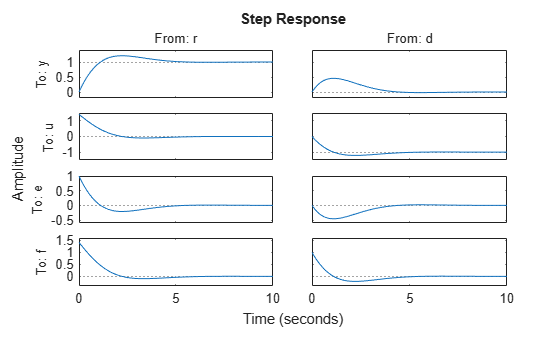Documentation

# imp2exp

Convert implicit linear relationship to explicit input-output relation

## Syntax

``B = imp2exp(A,yidx,uidx)``

## Description

example

````B = imp2exp(A,yidx,uidx)` transforms a linear constraint between variables `y` and `u` of the form `A(:,[yidx;uidx])*[y;u] = 0` into an explicit input/output relationship `y = B*u`. The vectors `yidx` and `uidx` refer to the columns (inputs) of `A` as referenced by the explicit relationship for `B`.```

## Examples

collapse all

Consider two uncertain motor/generator constraints among 4 variables $\left[\begin{array}{c}V\\ I\\ T\\ W\end{array}\right]$, namely $\left[\begin{array}{cccc}1& -R& 0& -K\\ 0& -K& 1& 0\end{array}\right]*\left[\begin{array}{c}V\\ I\\ T\\ W\end{array}\right]=0$. Find the uncertain 2-by-2 matrix `B` so that $\left[\begin{array}{c}V\\ T\end{array}\right]=B*\left[\begin{array}{c}W\\ I\end{array}\right]$.

```R = ureal('R',1,'Percentage',[-10 40]); K = ureal('K',2e-3,'Percentage',[-30 30]); A = [1 -R 0 -K;0 -K 1 0]; Yidx = [1 3]; Uidx = [4 2]; B = imp2exp(A,Yidx,Uidx) ```
```B = Uncertain matrix with 2 rows and 2 columns. The uncertainty consists of the following blocks: K: Uncertain real, nominal = 0.002, variability = [-30,30]%, 2 occurrences R: Uncertain real, nominal = 1, variability = [-10,40]%, 1 occurrences Type "B.NominalValue" to see the nominal value, "get(B)" to see all properties, and "B.Uncertainty" to interact with the uncertain elements. ```

Consider a standard single-loop feedback connection of controller `C` and an uncertain plant `P`, described by the equations `e = r-y; u = Ce; f = d+u; y = Pf`.```P = tf(,[1 0]); C = tf([2*.707*1 1^2],[1 0]); A = [1 -1 0 0 0 -1;0 -C 1 0 0 0;0 0 -1 -1 1 0;0 0 0 0 -P 1]; OutputIndex = [6;3;2;5]; % [y;u;e;f] InputIndex = [1;4]; % [r;d] Sys = imp2exp(A,OutputIndex,InputIndex); Sys.InputName = {'r';'d'}; Sys.OutputName = {'y';'u';'e';'f'};```
`pole(Sys)`
```ans = 4×1 complex -0.7070 + 0.7072i -0.7070 - 0.7072i -0.7070 + 0.7072i -0.7070 - 0.7072i ```
`stepplot(Sys)`## Input Arguments

collapse all

Implicit system, specified as a static, or dynamic input/output model. `A` can be:

The number of rows in model `A` must equal the length of the index vector `yidx`.

Indices to partition the model channel into output signals, specified as a vector. The index vectors `yidx` and `uidx` specify how to partition the input channels of `A` into `y` and `u` signals, respectively.

If `[yidx,uidx]` does not include all inputs of `A`, `imp2exp` excludes the missing `y` channels from the output model `B`. In such cases, `imp2exp` retains only a subset `B(I,:)` of the outputs/rows of `B` and does not affect how the explicit model `B` is computed.

Indices to partition the model channel into input signals, specified as a vector. The index vectors `yidx` and `uidx` specify how to partition the input channels of `A` into `y` and `u` signals, respectively.

## Output Arguments

collapse all

Explicit system, returned as a static, or dynamic input/output model. The output explicit model `B` is of the same subclass as model `A`. For instance, if `A` is specified as an `ss` model, then `imp2exp` also returns `B` as an `ss` model.